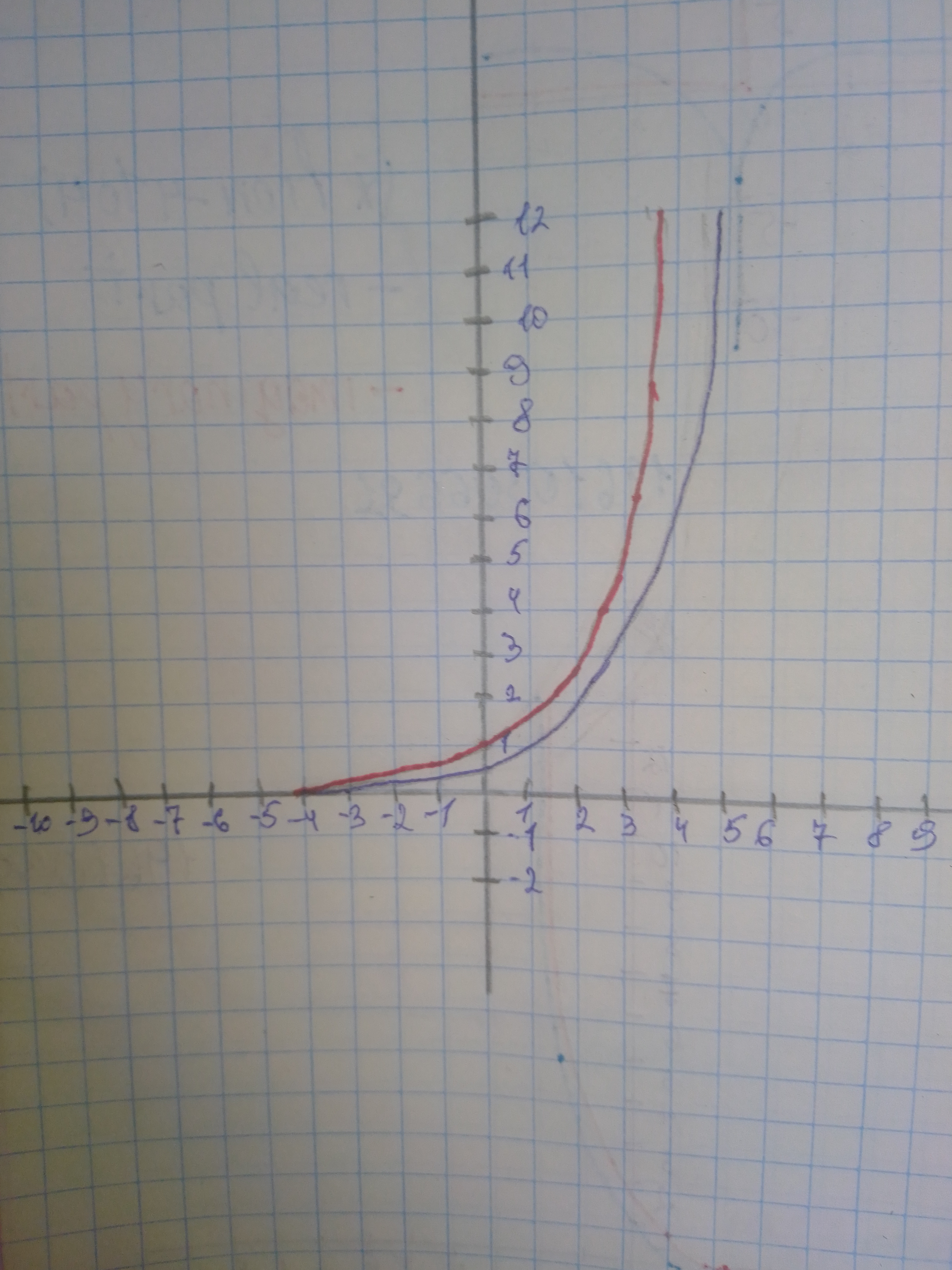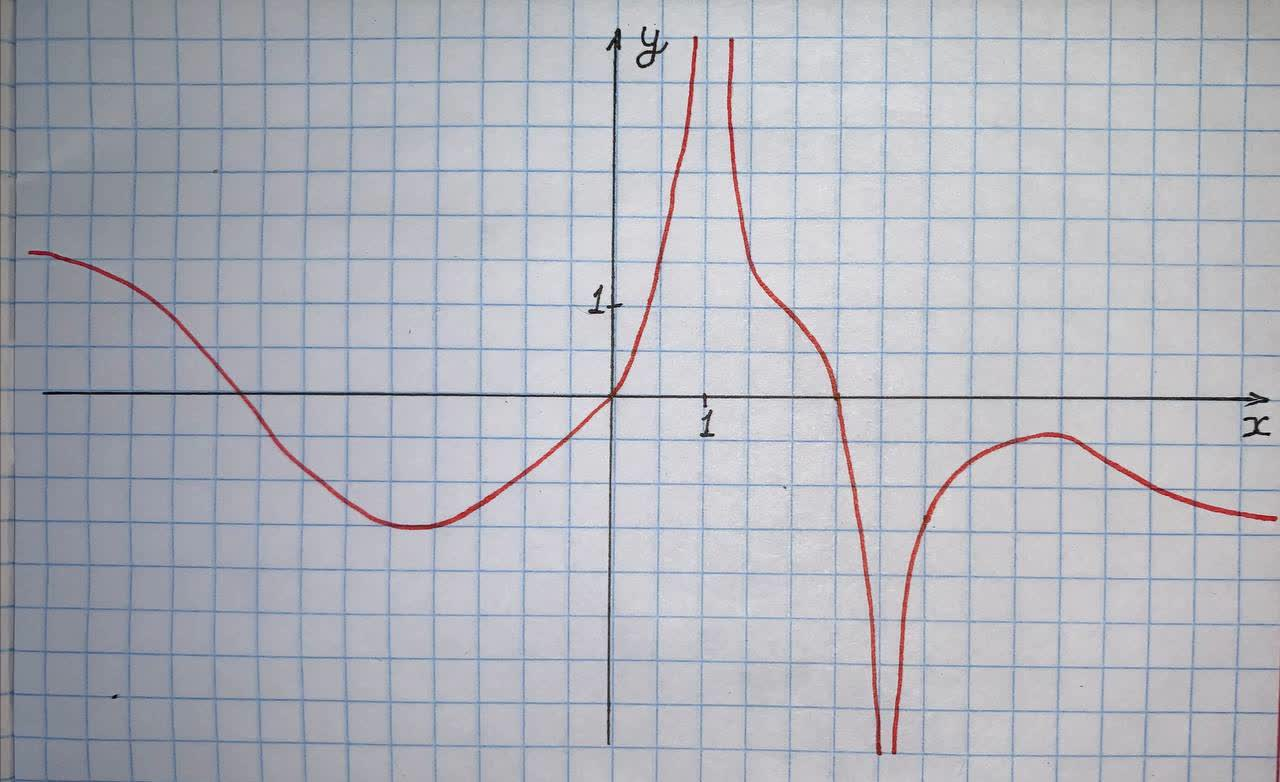Ask question

# Graph f and g in the same rectangular coordinate system. Use transformations of the graph f of to obtain the graph of g. Graph and give equations of all asymptotes. Use the graphs to determine each function's domain and range. f(x)=2^{x} text{and} g(x)=2^{x-1}# Graph f and g in the same rectangular coordinate system. Use transformations of the graph f of to obtain the graph of g. Graph and give equations of all asymptotes. Use the graphs to determine each function's domain and range. f(x)=2^{x} text{and} g(x)=2^{x-1}

Question
Transformations of functionsasked 2020-11-23
Graph f and g in the same rectangular coordinate system. Use transformations of the graph f of to obtain the graph of g. Graph and give equations of all asymptotes. Use the graphs to determine each function's domain and range. $$\displaystyle{f{{\left({x}\right)}}}={2}^{{{x}}}\text{and}{g{{\left({x}\right)}}}={2}^{{{x}-{1}}}$$

## Answers (1)2020-11-24

Step 1 $$\displaystyle{f{{\left({x}\right)}}}={2}^{{{x}}}\text{and}{g{{\left({x}\right)}}}={2}^{{{x}-{1}}}$$ Graph of g(x) can be obtained by translating the graph of f(x) by 1 unit tp the right along the x-axis. Domain of f(x) is $$\displaystyle{x}\in{\left(-\infty,\infty\right)}$$ Range of f(x) is $$\displaystyle{\left({0},\infty\right)}$$ Asympote for the graph is $$\displaystyle{y}={0}$$ Domain of $$\displaystyle{g{{\left({x}\right)}}}={x}\in{\left(-\infty,\infty\right)}$$ Range of PSKg(x) = (0, \infty) Asympote for the graph is $$\displaystyle{y}={0}$$### Relevant Questionsasked 2021-02-10
Graph f and g in the same rectangular coordinate system. Use transformations of the graph of f to obtain the graph of g. Graph and give equations of all asymptotes. Use the graphs to determine each function's domain and range. $$\displaystyle{f{{\left({x}\right)}}}={3}^{{{x}}}={3}^{{{x}}}\ -\ {1}$$asked 2020-12-24
Begin by graphing
$$\displaystyle{f{{\left({x}\right)}}}={2}^{{{x}}}.$$
Then use transformations of this graph to graph the given function. Be sure to graph and give equations of the asymptotes. Use the graphs to determine each function's domain and range. If applicable, use a graphing utility to confirm your hand-drawn graphs.
$$\displaystyle{g{{\left({x}\right)}}}={2}^{{{x}\ +\ {2}}}$$asked 2021-02-08
Begin by graphing
$$\displaystyle{f{{\left({x}\right)}}}={2}^{{{x}}}$$
Then use transformations of this graph to graph the given function. Be sure to graph and give equations of the asymptotes. Use the graphs to determine each function's domain and range. If applicable, use a graphing utility to confirm your hand-drawn graphs.
$$\displaystyle{g{{\left({x}\right)}}}=-{2}^{{{x}}}$$asked 2021-06-06
For each of the following functions f (x) and g(x), express g(x) in the form a: f (x + b) + c for some values a,b and c, and hence describe a sequence of horizontal and vertical transformations which map f(x) to g(x).
$$\displaystyle{f{{\left({x}\right)}}}={x}^{{2}}-{2},{g{{\left({x}\right)}}}={2}+{8}{x}-{4}{x}^{{2}}$$asked 2021-01-15
Begin by graphing
$$\displaystyle{f{{\left({x}\right)}}}={2}^{{{2}}}$$
Then use transformations of this graph to graph the given function. Be sure to graph and give equations of the asymptotes. Use the graphs to determine each function's domain and range. If applicable, use a graphing utility to confirm your hand-drawn graphs.
$$\displaystyle{g{{\left({x}\right)}}}={2}^{{-{x}}}$$asked 2020-12-15
In the following items, you will analyze how several transformations affect the graph of the function $$\displaystyle{f{{\left({x}\right)}}}={\frac{{{1}}}{{{x}}}}$$. Investigate the graphs of $$\displaystyle{f{{\left({x}\right)}}}={\frac{{{1}}}{{{x}}}},{g{{\left({x}\right)}}}={f{{\left({x}\right)}}}={\frac{{{1}}}{{{x}+{2}}}},{h}{\left({x}\right)}={\frac{{{1}}}{{{x}-{2}}}},{p}{\left({x}\right)}={\frac{{{1}}}{{{x}-{4}}}}\ \text{and}\ {z}{\left({x}\right)}={\frac{{{1}}}{{{x}^{{{2}}}+{1}}}}$$. If you use a graphing calculator, select a viewing window $$\displaystyle\pm{23.5}$$ for x and $$\displaystyle\pm{15.5}$$ for y. At what values in the domain did vertical asymptotes occur for each of the functions? Explain why the vertical asymptotes occur at these values.asked 2021-01-10
Begin by graphing $$\displaystyle{f{{\left({x}\right)}}}=\ {{\log}_{{{2}}}{x}}$$ Then use transformations of this graph to graph the given function. What is the vertical asymptote? Use the graphs to determine each function's domain and range. $$\displaystyle{g{{\left({x}\right)}}}=\ -{2}\ {{\log}_{{{2}}}{x}}$$asked 2021-01-10
Begin by graphing
$$\displaystyle{f{{\left({x}\right)}}}={{\log}_{{{2}}}{x}}$$
Then use transformations of this graph to graph the given function. What is the vertical asymptote? Use the graphs to determine each function s domain and range.
$$\displaystyle{g{{\left({x}\right)}}}={\frac{{{1}}}{{{2}}}}{{\log}_{{{2}}}{x}}$$asked 2021-05-13
For the function f whose graph is given, state the following.(a) $$\lim_{x \rightarrow \infty} f(x)$$
b) $$\lim_{x \rightarrow -\infty} f(x)$$
(c) $$\lim_{x \rightarrow 1} f(x)$$
(d) $$\lim_{x \rightarrow 3} f(x)$$
(e) the equations of the asymptotes
Vertical:- ?
Horizontal:-?asked 2021-01-28
Begin by graphing $$\displaystyle{f{{\left({x}\right)}}}={{\log}_{{{2}}}{x}}$$ Then use transformations of this graph to graph the given function. What is the vertical asymptote? Use the graphs to determine each functions domain and range. $$\displaystyle{g{{\left({x}\right)}}}=\ -{2}{{\log}_{{{2}}}{x}}$$
...